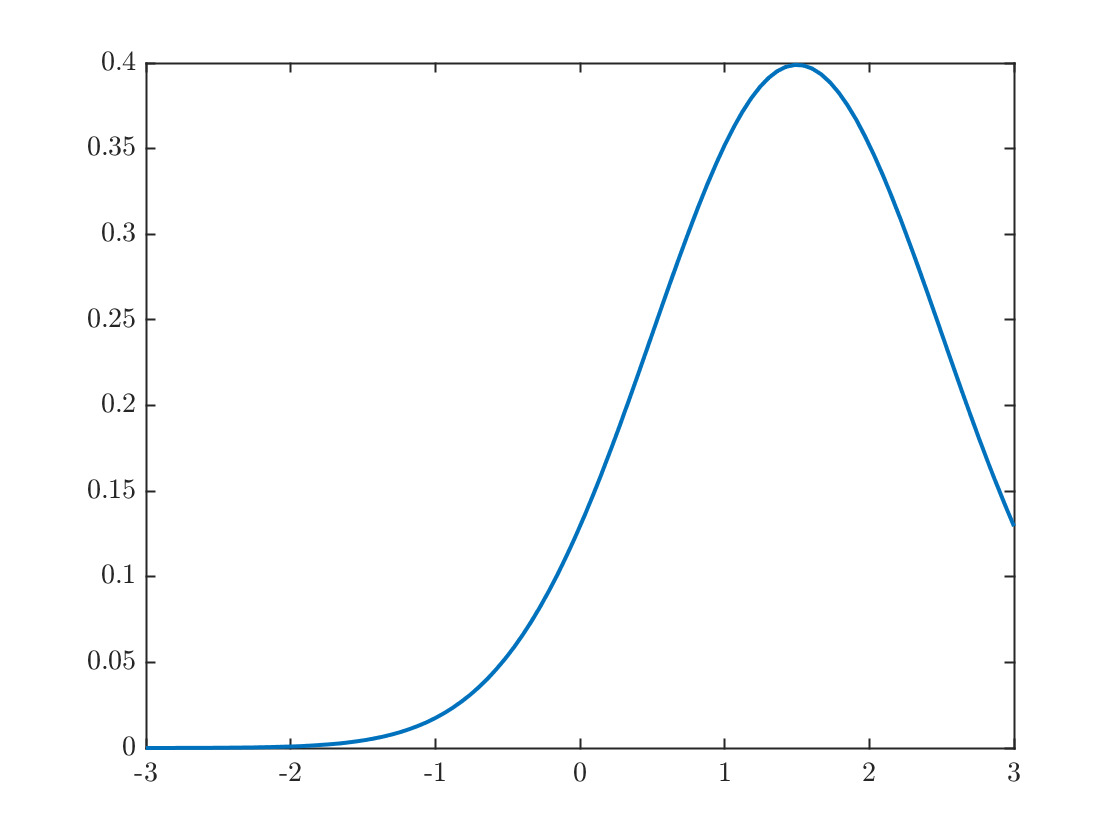# Math 222 MATLAB Supplementary lecture

## Part 2: Defining your own functions

MATLAB has lots of built-in functions like sin, exp, and abs, for defining the sine, exponential and absolute value functions, but to build a proper Euler method program, we need to be able to define our own.
Here's an example of a function definition. The normal distribution with mean μ and variance σ is defined aswhich we can put into a function as
function y = gaussian(x,mu,sigma)
y = exp(-1/2 * ( (x-mu)/sigma).^2) / sigma / sqrt(2*pi);
end
The above three lines are only example code, but they are repeated at the bottom of this live script, and those lines are callable code. To define a function that you'd like to be able to reuse, you could save this to a file called gaussian.m instead of simply placing it inside another program.
We can call this with the command
x=linspace(-3,3);
mu = 1.5;
sigma=1;
y= gaussian(x,mu,sigma);
plot(x,y)A function can also have multiple outputs. For example
function [s,p]=sumprod(x1,x2)
s = x1+x2;
p = x1*x2;
end
Finally, simple functions with one output argumet can be defined quickly using the anonymous function syntax.
gaussian = @(x,mu,sigma) exp(-1/2 * ( (x-mu)/sigma).^2) / sigma / sqrt(2*pi);
This is called exactly like any other function. Anonymous functions can only have one output argument, so we can't write the sumprod example above this way.

## Function definition

function y = gaussian(x,mu,sigma)
y = exp(-1/2 * ( (x-mu)/sigma).^2) / sigma / sqrt(2*pi);
end